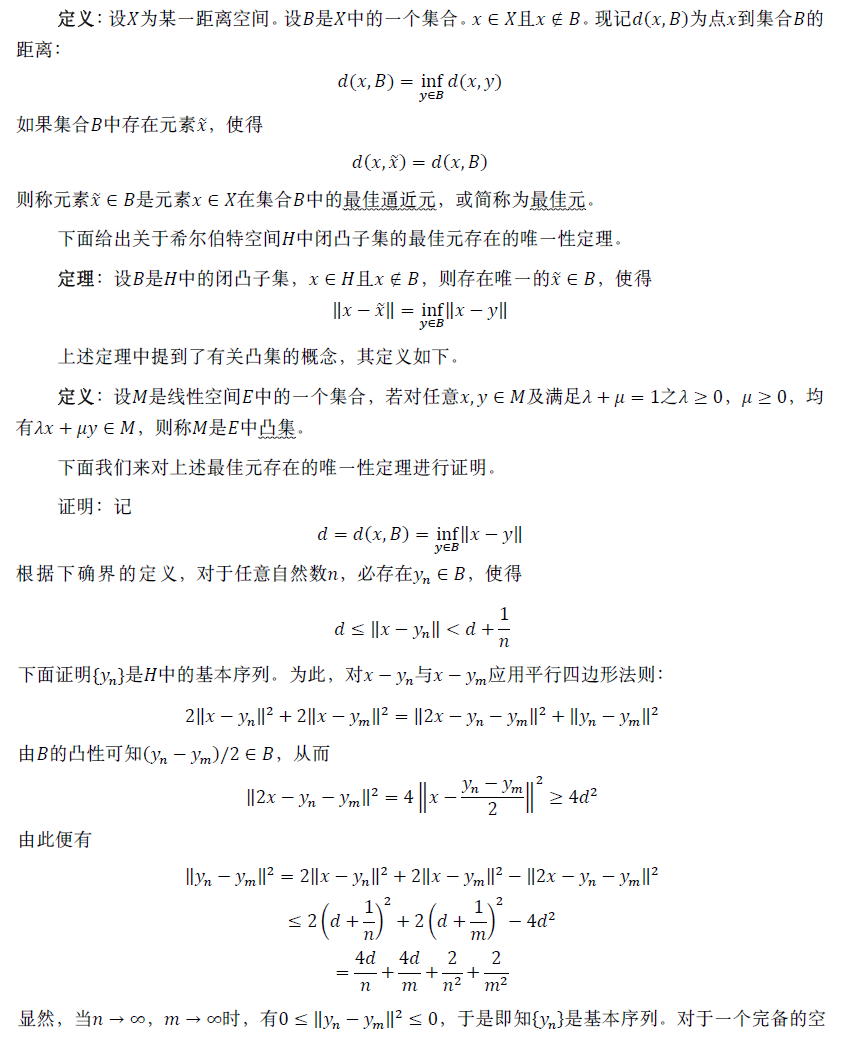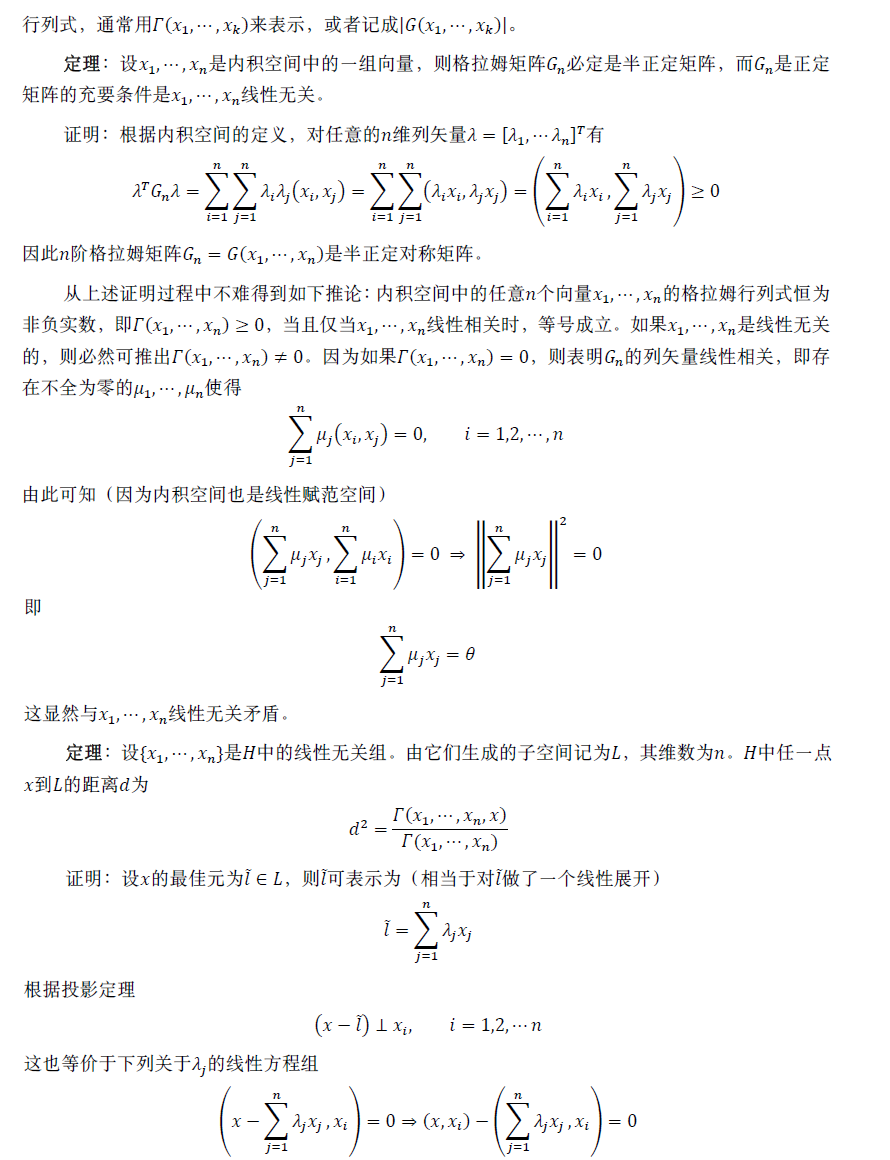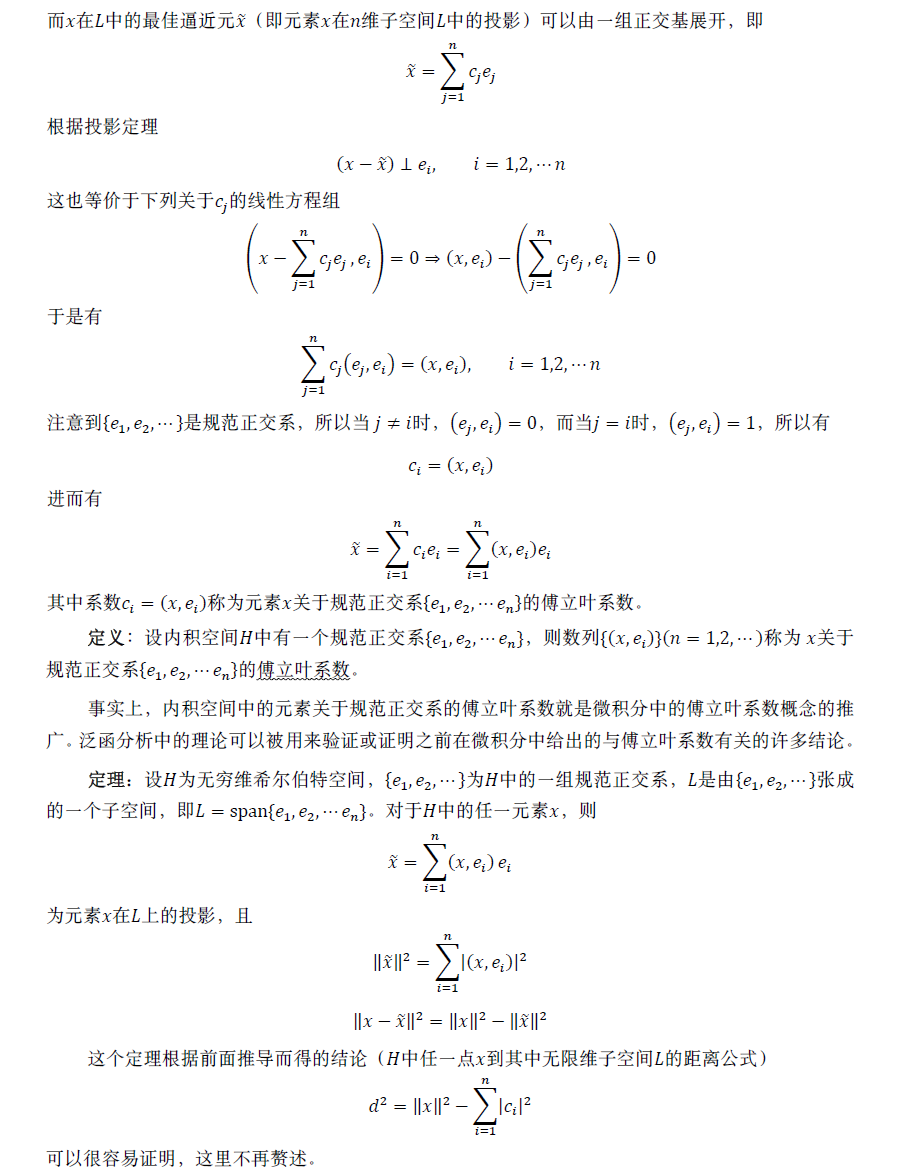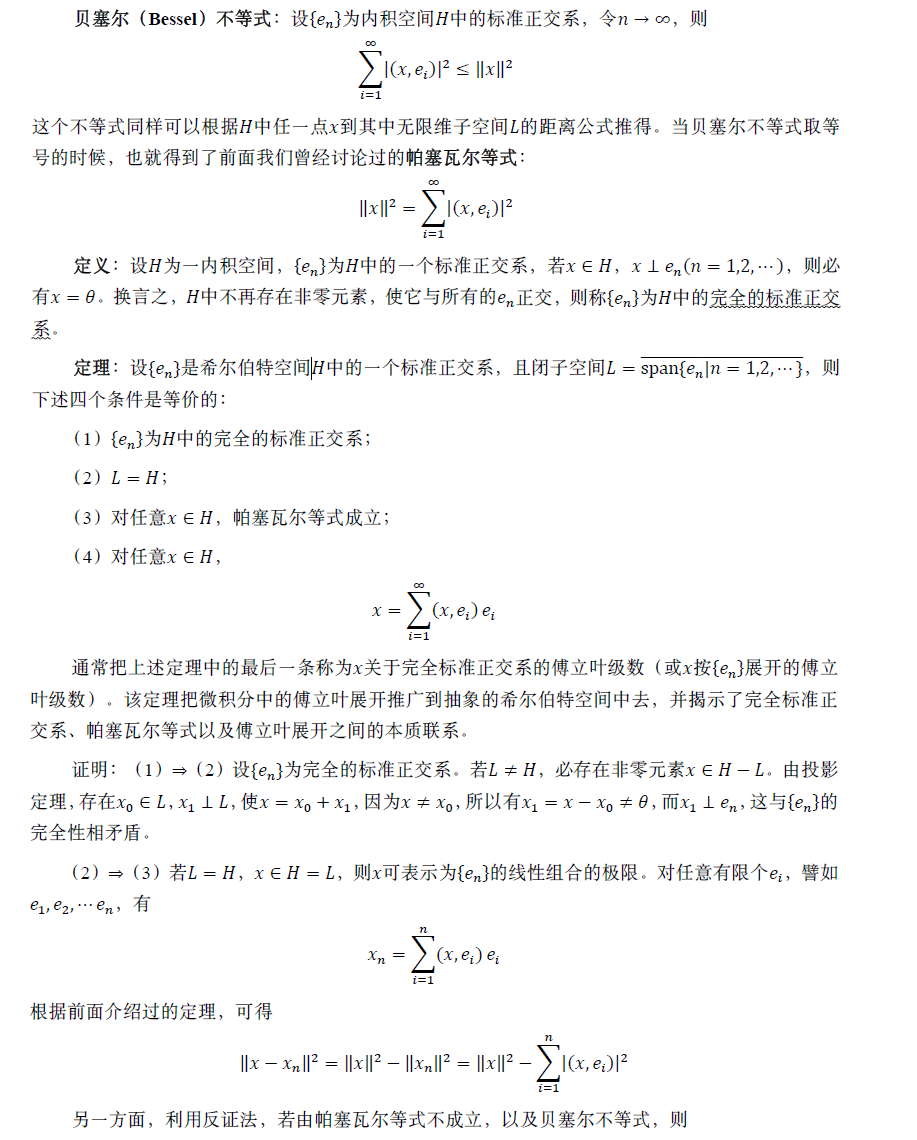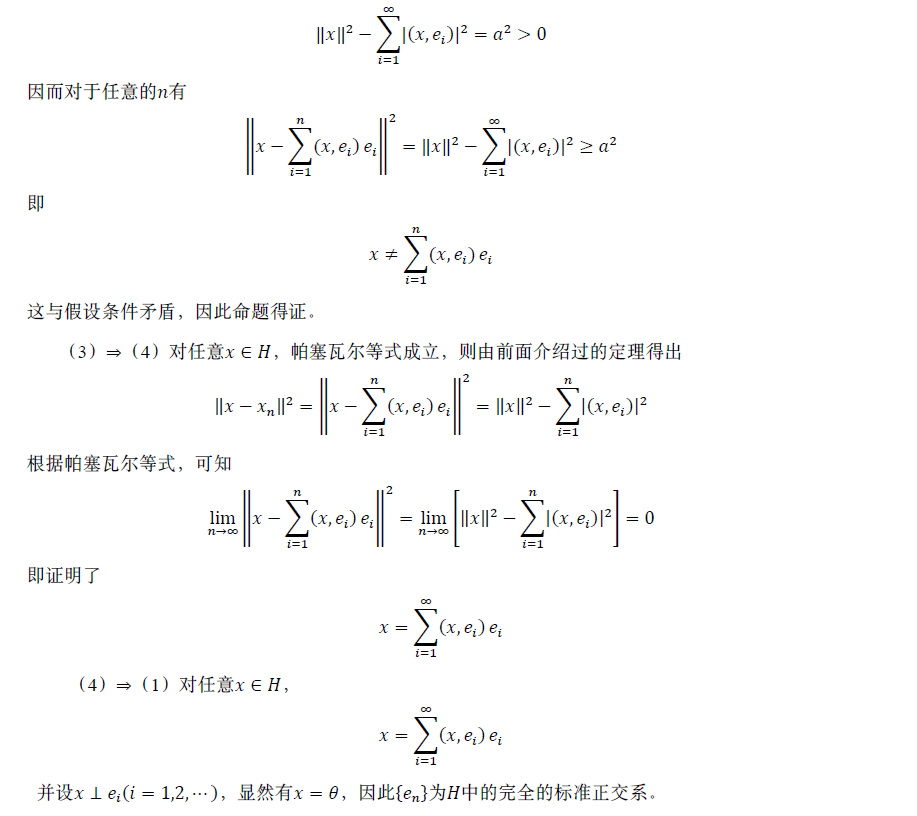2017-05-06 00:42:13 baimafujinji 阅读数 9297
• ###### MATLAB图像处理

全面系统的学习MATLAB在图像处理中的应用

19905 人正在学习 去看看 魏伟

• 基于暗通道的图像去雾实现
• 对比度有限的自适应直方图均衡（CLAHE）
• 自适应直方图均衡（AHE）
• 基于小波变换的图像融合（失焦图像修复）
• 基于泊松方程的泊松融合算法（基于迭代）
• 基于泊松方程的泊松融合算法（有限差分方法解偏微分方程）
• 基于主成分提取（PCA）的图像压缩

## P270

i=double(imread('vase.tif'));
[C,S]=wavedec2(i,2,'db1');
a2=appcoef2(C,S,'db1',2);
dh1=detcoef2('h',C,S,1);
dv1=detcoef2('v',C,S,1);
dd1=detcoef2('d',C,S,1);
dh2=detcoef2('h',C,S,2);
dv2=detcoef2('v',C,S,2);
dd2=detcoef2('d',C,S,2);
[x,y]=size(i);
img = zeros(x,y);
img(1:x/4,1:y/4) =im2uint8(mat2gray(a2));
img(((x/4)+1):y/2,1:y/4) = im2uint8(mat2gray(dv2));
img(((x/4)+1):x/2,1:y/4) = im2uint8(mat2gray(dv2));
img(1:x/4,((y/4)+1):y/2) = im2uint8(mat2gray(dh2));
img(((x/4)+1):x/2,((y/4)+1):y/2) = im2uint8(mat2gray(dd2));
img(((x/2)+1):x,1:y/2) = im2uint8(mat2gray(dv1));
img(1:x/2,((y/2)+1):y) = im2uint8(mat2gray(dh1));
img(((x/2)+1):x,((y/2)+1):y) = im2uint8(mat2gray(dd1));
imshow(img,[]);


## P272

X1 = imread('cathe1.bmp');
XFUS = wfusimg(X1,X2,'sym4',5,'mean','max');
imshow(XFUS,[]);


## P273

X1 = imread('cathe1.bmp');
M1 = double(X1) / 256;
M2 = double(X2) / 256;
N = 4;
wtype = 'sym4';
[c0,s0] = wavedec2(M1, N, wtype);
[c1,s1] = wavedec2(M2, N, wtype);
length = size(c1);
Coef_Fusion = zeros(1,length(2));
%低频系数的处理，取平均值
Coef_Fusion(1:s1(1,1)) = (c0(1:s1(1,1))+c1(1:s1(1,1)))/2;
%处理高频系数，取绝对值大者，这里用到了矩阵乘法
MM1 = c0(s1(1,1)+1:length(2));
MM2 = c1(s1(1,1)+1:length(2));
mm = (abs(MM1)) > (abs(MM2));
Y  = (mm.*MM1) + ((~mm).*MM2);
Coef_Fusion(s1(1,1)+1:length(2)) = Y;
%重构
Y = waverec2(Coef_Fusion,s0,wtype);
imshow(Y,[]);


## P274

I = imread('noise_lena.bmp');
[thr,sorh,keepapp] = ddencmp('den','wv',I);
de_I = wdencmp('gbl',I,'sym4',2,thr,sorh,keepapp);
imwrite(im2uint8(mat2gray(de_I)), 'denoise_lena.bmp');


## P298

I = imread('baboon.bmp');
I1 = double(I);
myFun1 = @(block_struct)T*block_struct.data*T/64;
H = blockproc(I1, [8 8], myFun1);
H(abs(H)<3.5)=0;
myFun2 = @(block_struct)T*block_struct.data*T;
I2 = blockproc(H, [8 8], myFun2);
subplot(121), imshow(I1,[]), title('original image');
subplot(122), imshow(I2,[]), title('zipped image');


## P299

I = imread('baboon.bmp');
I1 = double(I);
[m n] =size(I);
sizi = 8;
num = 16;
%分块进行离散沃尔什变换
myFun1 = @(block_struct)T*block_struct.data*T/(sizi.^2);
hdcoe = blockproc(I1, [sizi, sizi], myFun1);
%重新排列系数
coe = im2col(hdcoe,  [sizi, sizi], 'distinct');
coe_t = abs(coe);
[Y, ind] = sort(coe_t);
%舍去绝对值较小的系数
[m_c, n_c] = size(coe);
for i = 1:n_c
coe(ind(1:num, i), i)=0;
end
%重建图像
re_hdcoe = col2im(coe, [sizi, sizi], [m, n], 'distinct');
myFun2 = @(block_struct)T*block_struct.data*T;
re_s = blockproc(re_hdcoe, [sizi, sizi], myFun2);
subplot(121), imshow(I1,[]), title('original image');
subplot(122), imshow(re_s,[]), title('compressed image');


## P307

I = imread('baboon.bmp');
x = double(I)/255;
[m,n]=size(x);
y =[];
%拆解图像
for i = 1:m/8;
for j = 1:n/8;
ii = (i-1)*8+1;
jj = (j-1)*8+1;
y_app = reshape(x(ii:ii+7,jj:jj+7),1,64);
y=[y;y_app];
end
end

%KL变换
[COEFF,SCORE,latent] = princomp(y);
kl = y * COEFF;

kl1 = kl;
kl2 = kl;
kl3 = kl;

%置零压缩过程
kl1(:, 33:64)=0;
kl2(:, 17:64)=0;
kl3(:, 9:64)=0;

%KL逆变换
kl_i = kl*COEFF';
kl1_i = kl1*COEFF';
kl2_i = kl2*COEFF';
kl3_i = kl3*COEFF';

image = ones(256,256);
image1 = ones(256,256);
image2 = ones(256,256);
image3 = ones(256,256);

k=1;
%重组图像
for i = 1:m/8;
for j = 1:n/8;

y = reshape(kl_i(k, 1:64),8,8);
y1 = reshape(kl1_i(k, 1:64),8,8);
y2 = reshape(kl2_i(k, 1:64),8,8);
y3 = reshape(kl3_i(k, 1:64),8,8);

ii = (i-1)*8+1;
jj = (j-1)*8+1;

image(ii:ii+7,jj:jj+7) = y;
image1(ii:ii+7,jj:jj+7) = y1;
image2(ii:ii+7,jj:jj+7) = y2;
image3(ii:ii+7,jj:jj+7) = y3;

k=k+1;
end
end


##P356-1

mountains = double(imread('./img/mountain.jpg'));
sizeSrc = size(mountains);
sizeDst = size(moon);

+ moon(3:sizeDst(1),2:sizeDst(2)-1,:)...
+ moon(2:sizeDst(1)-1,1:sizeDst(2)-2,:)...
+ moon(2:sizeDst(1)-1,3:sizeDst(2),:)...
- 4*moon(2:sizeDst(1)-1,2:sizeDst(2)-1,:);


## P356-2

Lap = [0, 1, 0;1, -4, 1;0, 1, 0];

I1 = conv2(double(moon(:,:,1)), double(Lap));
I2 = conv2(double(moon(:,:,2)), double(Lap));
I3 = conv2(double(moon(:,:,3)), double(Lap));


## P357

dstX = 350;dstY = 100;
rebuilt = mountains(dstY:dstY+sizeDst(1)-1,dstX:dstX+sizeDst(2)-1,:);

for n = [1:1000]
rebuilt(2:2:sizeDst(1)-1,2:2:sizeDst(2)-1,:)= ...
(rebuilt(1:2:sizeDst(1)-2 , 2:2:sizeDst(2)-1,:)...
+rebuilt(3:2:sizeDst(1) , 2:2:sizeDst(2)-1,:)...
+rebuilt(2:2:sizeDst(1)-1 , 1:2:sizeDst(2)-2,:)...
+rebuilt(2:2:sizeDst(1)-1 , 3:2:sizeDst(2),:)...
rebuilt(3:2:sizeDst(1)-1,3:2:sizeDst(2)-1,:)= ...
(rebuilt(2:2:sizeDst(1)-2 , 3:2:sizeDst(2)-1,:)...
+rebuilt(4:2:sizeDst(1) , 3:2:sizeDst(2)-1,:)...
+rebuilt(3:2:sizeDst(1)-1 , 2:2:sizeDst(2)-2,:)...
+rebuilt(3:2:sizeDst(1)-1 , 4:2:sizeDst(2),:)...
rebuilt(3:2:sizeDst(1)-1,2:2:sizeDst(2)-1,:)= ...
(rebuilt(2:2:sizeDst(1)-2 , 2:2:sizeDst(2)-1,:)...
+rebuilt(4:2:sizeDst(1) , 2:2:sizeDst(2)-1,:)...
+rebuilt(3:2:sizeDst(1)-1 , 1:2:sizeDst(2)-2,:)...
+rebuilt(3:2:sizeDst(1)-1 , 3:2:sizeDst(2),:)...
rebuilt(2:2:sizeDst(1)-1 , 3:2:sizeDst(2)-1,:)= ...
(rebuilt(1:2:sizeDst(1)-2 , 3:2:sizeDst(2)-1,:)...
+rebuilt(3:2:sizeDst(1) , 3:2:sizeDst(2)-1,:)...
+rebuilt(2:2:sizeDst(1)-1 , 2:2:sizeDst(2)-2,:)...
+rebuilt(2:2:sizeDst(1)-1 , 4:2:sizeDst(2),:)...
end

mountains(dstY:sizeDst(1)+dstY-1,dstX:sizeDst(2)+dstX-1,:) = rebuilt;
figure,imshow(uint8(mountains));


## P360-P361

TargetImg   = imread('pool-target.jpg');

figure, imshow(SourceImg), axis image
hold on
for k = 1:length(SrcBoundry)
boundary = SrcBoundry{k};
plot(boundary(:,2), boundary(:,1), 'r', 'LineWidth', 2)
end
title('Source image intended area for cutting from');

position_in_target = [10, 225];%xy
[TargetRows, TargetCols, ~] = size(TargetImg);
start_pos = [min(col), min(row)];
end_pos  = [max(col), max(row)];
frame_size = end_pos - start_pos;

if (frame_size(1) + position_in_target(1) > TargetCols)
position_in_target(1) = TargetCols - frame_size(1);
end

if (frame_size(2) + position_in_target(2) > TargetRows)
position_in_target(2) = TargetRows - frame_size(2);
end

position_in_target(2), col-start_pos(1)+position_in_target(1))) = 1;

figure, imshow(TargetImg), axis image
hold on
for k = 1:length(TargBoundry)
boundary = TargBoundry{k};
plot(boundary(:,2), boundary(:,1), 'r', 'LineWidth', 1)
end


## P362

templt = [0 -1 0; -1 4 -1; 0 -1 0];
LaplacianSource = imfilter(double(SourceImg), templt, 'replicate');
VR = LaplacianSource(:, :, 1);
VG = LaplacianSource(:, :, 2);
VB = LaplacianSource(:, :, 3);

TargetImgR = double(TargetImg(:, :, 1));
TargetImgG = double(TargetImg(:, :, 2));
TargetImgB = double(TargetImg(:, :, 3));

TargetImgNew = cat(3, TargetImgR, TargetImgG, TargetImgB);
figure, imagesc(uint8(TargetImgNew)), axis image;

ResultImg = cat(3, ResultImgR, ResultImgG, ResultImgB);

%% Show the final results
figure;
imshow(uint8(ResultImg));


## P393

image = imread('Unequalized_Hawkes_Bay_NZ.jpg');
Img = rgb2gray(image);
[height,width]=size(Img);

NumPixel = zeros(1,256);%统计各灰度数目，共256个灰度级
for i = 1:height
for j = 1: width
%对应灰度值像素点数量增加一
%因为NumPixel的下标是从1开始，但是图像像素的取值范围是0~255，
%所以用NumPixel(Img(i,j) + 1)
NumPixel(Img(i,j) + 1) = NumPixel(Img(i,j) + 1) + 1;
end
end

ProbPixel = zeros(1,256);
for i = 1:256
ProbPixel(i) = NumPixel(i) / (height * width * 1.0);
end

CumuPixel = cumsum(ProbPixel);
CumuPixel = uint8(255 .* CumuPixel + 0.5);

for i = 1:height
for j = 1: width
Img(i,j) = CumuPixel(Img(i,j));
end
end

imshow(Img)


## P395-1

a = imread('couple.tiff');
R = a(:,:,1);
G = a(:,:,2);
B = a(:,:,3);

R = histeq(R, 256);
G = histeq(G, 256);
B = histeq(B, 256);

a(:,:,1) = R;
a(:,:,2) = G;
a(:,:,3) = B;
imshow(a)


## P395-2

Img = imread('couple.tiff');
hsvImg = rgb2hsv(Img);
V = hsvImg(:,:,3);
[height,width] = size(V);

V = uint8(V*255);
NumPixel = zeros(1,256);
for i = 1:height
for j = 1: width
NumPixel(V(i,j) + 1) = NumPixel(V(i,j) + 1) + 1;
end
end

ProbPixel = zeros(1,256);
for i = 1:256
ProbPixel(i) = NumPixel(i) / (height * width * 1.0);
end

CumuPixel = cumsum(ProbPixel);
CumuPixel = uint8(255 .* CumuPixel + 0.5);

for i = 1:height
for j = 1: width
if V(i,j)==0
V(i,j) = CumuPixel(V(i,j)+1);
else
V(i,j) = CumuPixel(V(i,j));
end
end
end

V = im2double(V);
hsvImg(:,:,3) = V;
outputImg = hsv2rgb(hsvImg);
imshow(outputImg);


## P397

img = imread('space.jpg');
rimg = img(:,:,1);
gimg = img(:,:,2);
bimg = img(:,:,3);
result = cat(3, resultr, resultg, resultb);
imshow(result);


## P398-1

clear;
cform2lab = makecform('srgb2lab');
LAB = applycform(img, cform2lab);
L = LAB(:,:,1);
cform2srgb = makecform('lab2srgb');
J = applycform(LAB, cform2srgb);
imshow(J);


## P398-2

clc;
clear all;
[h,w] = size(Img);
minV = double(min(min(Img)));
maxV = double(max(max(Img)));
imshow(Img);


## P399

NrX = 8;
NrY = 4;
HSize = ceil(h/NrY);
WSize = ceil(w/NrX);

deltay = NrY*HSize - h;
deltax = NrX*WSize - w;

tmpImg = zeros(h+deltay,w+deltax);
tmpImg(1:h,1:w) = Img;

new_w = w + deltax;
new_h = h + deltay;
NrPixels = WSize * WSize;

% NrBins - Number of greybins for histogram ("dynamic range")
NrBins = 256;

LUT = zeros(maxV+1,1);

for i=minV:maxV
LUT(i+1) = fix(i - minV);%i+1
end

Bin = zeros(new_h, new_w);
for m = 1 : new_h
for n = 1 : new_w
Bin(m,n) = 1 + LUT(tmpImg(m,n) + 1);
end
end

Hist = zeros(NrY, NrX, 256);
for i=1:NrY
for j=1:NrX
tmp = uint8(Bin(1+(i-1)*HSize:i*HSize, 1+(j-1)*WSize:j*WSize));
%tmp = tmpImg(1+(i-1)*HSize:i*HSize,1+(j-1)*WSize:j*WSize);
[Hist(i, j, :), x] = imhist(tmp, 256);
end
end

Hist = circshift(Hist,[0, 0, -1]);

ClipLimit = 2.5;
ClipLimit = max(1,ClipLimit * HSize * WSize/NrBins);
Hist = clipHistogram(Hist,NrBins,ClipLimit,NrY,NrX);
Map=mapHistogram(Hist, minV, maxV, NrBins, NrPixels, NrY, NrX);

yI = 1;
for i = 1:NrY+1
if i == 1
subY = floor(HSize/2);
yU = 1;
yB = 1;
elseif i == NrY+1
subY = floor(HSize/2);
yU = NrY;
yB = NrY;
else
subY = HSize;
yU = i - 1;
yB = i;
end
xI = 1;
for j = 1:NrX+1
if j == 1
subX = floor(WSize/2);
xL = 1;
xR = 1;
elseif j == NrX+1
subX = floor(WSize/2);
xL = NrX;
xR = NrX;
else
subX = WSize;
xL = j - 1;
xR = j;
end
UL = Map(yU,xL,:);
UR = Map(yU,xR,:);
BL = Map(yB,xL,:);
BR = Map(yB,xR,:);
subImage = Bin(yI:yI+subY-1,xI:xI+subX-1);

%%%%%%%%%%%%%%%%%%%%%%%%%%%%%%%%%%%%%%%%%%%%%%%%%%%%%%%%%%
sImage = zeros(size(subImage));
num = subY * subX;
for i = 0:subY - 1
inverseI = subY - i;
for j = 0:subX - 1
inverseJ = subX - j;
val = subImage(i+1,j+1);
sImage(i+1, j+1)=(inverseI*(inverseJ*UL(val)+j*UR(val))...
+ i*(inverseJ*BL(val)+j*BR(val)))/num;
end
end
%%%%%%%%%%%%%%%%%%%%%%%%%%%%%%%%%%%%%%%%%%%%%%%%%%%%%%%%%%

output(yI:yI+subY-1, xI:xI+subX-1) = sImage;
xI = xI + subX;
end
yI = yI + subY;
end

output = output(1:h, 1:w);
figure, imshow(output, []);


## P404

img = rgb2gray(imread('theatre.jpg'));
[hgram, x] = imhist(img_ref);
J = histeq(img, hgram);
subplot(2,3,1), imshow(img), title('original image');
subplot(2,3,4), imhist(img), title('original image');
subplot(2,3,2), imshow(img_ref), title('reference image');
subplot(2,3,5), imhist(img_ref), title('reference image');
subplot(2,3,3), imshow(J), title('output image');
subplot(2,3,6), imhist(J), title('output image');


## P409

%求一幅图像的暗通道图，窗口大小为15*15
imageRGB = double(imageRGB);
imageRGB = imageRGB./255;
dark = darkChannel(imageRGB);

% 选取暗通道图中最亮的0.1%像素，从而求得大气光
[m, n, ~] = size(imageRGB);
imsize = m * n;
numpx = floor(imsize/1000);
JDarkVec = reshape(dark,imsize,1);
ImVec = reshape(imageRGB,imsize,3);

[JDarkVec, indices] = sort(JDarkVec);
indices = indices(imsize-numpx+1:end);

atmSum = zeros(1,3);
for ind = 1:numpx
atmSum = atmSum + ImVec(indices(ind),:);
end

atmospheric = atmSum / numpx;

%求解透射率，并通过omega参数来选择保留一定程度的雾霾，以免损坏真实感
omega = 0.95;
im = zeros(size(imageRGB));

for ind = 1:3
im(:,:,ind) = imageRGB(:,:,ind)./atmospheric(ind);
end

dark_2 = darkChannel(im);
t = 1-omega*dark_2;

%通过导向滤波来获得更为精细的透射图
r = 60;
eps = 10^-6;
refined_t = guidedfilter_color(imageRGB, t, r, eps);


## P410

function dark = darkChannel(imRGB)

r=imRGB(:,:,1);
g=imRGB(:,:,2);
b=imRGB(:,:,3);

[m n] = size(r);
a = zeros(m,n);
for i = 1: m
for j = 1: n
a(i,j) = min(r(i,j), g(i,j));
a(i,j)= min(a(i,j), b(i,j));
end
end

d = ones(15,15);
fun = @(block_struct)min(min(block_struct.data))*d;
dark = blockproc(a, [15 15], fun);

dark = dark(1:m, 1:n);


## 本书源起2015-12-14 14:18:12 baimafujinji 阅读数 7193
• ###### MATLAB图像处理

全面系统的学习MATLAB在图像处理中的应用

19905 人正在学习 去看看 魏伟

http://blog.csdn.net/baimafujinji/article/details/48467225

1.1.3 函数的极限2015-09-15 15:10:15 baimafujinji 阅读数 37711
• ###### MATLAB图像处理

全面系统的学习MATLAB在图像处理中的应用

19905 人正在学习 去看看 魏伟

1.1  极限及其应用
1.1.1  数列的极限
1.1.2  级数的敛散
1.1.3  函数的极限
1.1.4  极限的应用

1.2  微分中值定理
1.2.1  罗尔中值定理
1.2.2  拉格朗日中值定理
1.2.3  柯西中值定理
1.2.4  泰勒公式

1.2.5  海塞矩阵与多元函数极值

1.3  向量代数与场论
1.3.1  牛顿-莱布尼茨公式
1.3.2  内积与外积
1.3.3  方向导数与梯度
1.3.4  曲线积分
1.3.5  格林公式
1.3.6  积分与路径无关条件
1.3.7  曲面积分
1.3.8  高斯公式与散度
1.3.9  斯托克斯公式与旋度

2.1  傅立叶级数展开
2.1.1  函数项级数的概念
2.1.2  函数项级数的性质
2.1.3  傅立叶级数的概念
2.1.4  傅立叶变换的由来
2.1.5  卷积定理及其证明

2.2  复变函数论初步
2.2.1  解析函数
2.2.2  复变积分
2.2.3  基本定理
2.2.4  级数展开

2.3  凸函数与詹森不等式

2.3.1  凸函数的概念

2.3.2  詹森不等式及其证明

2.3.3  詹森不等式的应用

2.4  常用经典数值解法

2.4.1  牛顿迭代法

2.4.2  雅各比迭代

2.4.3  高斯迭代法

2.4.4  托马斯算法

3.1  勒贝格积分理论
3.1.1  点集的勒贝格测度
3.1.2  可测函数及其性质
3.1.3  勒贝格积分的定义
3.1.4  积分序列极限定理
3.2  泛函与抽象空间
3.2.1  线性空间
3.2.2  距离空间
3.2.3  赋范空间
3.2.4  巴拿赫空间
3.2.5  内积空间
3.2.6  希尔伯特空间
3.2.7  索伯列夫空间
3.3  从泛函到变分法
3.3.1  理解泛函的概念
3.3.2  关于的变分概念
3.3.3  变分法的基本方程
3.3.4  理解哈密尔顿原理
3.3.5  等式约束下的变分
3.3.6  巴拿赫不动点定理
3.3.7  有界变差函数空间

4.1  概率论的基本概念

4.2  随机变量数字特征

4.2.1  期望

4.2.2  方差

4.2.3  矩与矩母函数

4.2.4  协方差与协方差矩阵

4.3  基本概率分布模型

4.3.1  离散概率分布

4.3.2  连续概率分布

4.4  概率论中的重要定理

4.4.1  大数定理

4.4.2  中央极限定理

4.5  随机采样

4.5.1  随机采样分布

4.5.2  蒙特卡洛采样

4.6  参数估计

4.7  假设检验

4.7.1  基本概念

4.7.2  两类错误

4.7.3  均值检验

4.8  极大似然估计

4.8.1  极大似然法的基本原理

4.8.2  求极大似然估计的方法

4.9  贝叶斯推断

4.9.1  先验概率与后验概率

4.9.2  共轭分布

5.1  图像编码的理论基础
5.1.1  率失真函数
5.1.2  香农下边界
5.1.3  无记忆高斯信源
5.1.4  有记忆高斯信源
5.2  子带编码基本原理
5.2.1  数字信号处理基础
5.2.2  多抽样率信号处理
5.2.3  图像信息子带分解
5.3  哈尔函数及其变换
5.3.1  哈尔函数的定义
5.3.2  哈尔函数的性质
5.3.3 酉矩阵与酉变换
5.3.4 二维离散线性变换
5.3.5 哈尔基函数
5.3.6 哈尔变换
5.4  小波及其数学原理
5.4.1  小波的历史
5.4.2  理解小波的概念
5.4.3  多分辨率分析
5.4.4  小波函数的构建
5.4.5  小波序列展开
5.4.6  离散小波变换
5.4.7  连续小波变换
5.4.8  小波的容许条件与基本特征
5.5  快速小波变换算法
5.5.1  快速小波正变换
5.5.2  快速小波逆变换
5.5.3  图像的小波变换

5.6  小波在图像处理中的应用

6.1  傅立叶变换
6.1.1  信号处理中的傅立叶变换

1. 连续时间，连续频率——傅立叶变换

2. 连续时间，离散频率——傅立叶级数

3. 离散时间，连续频率——序列的傅立叶变换

4. 离散时间，离散频率——离散的傅立叶变换

6.1.2  数字图像的傅立叶变换
6.1.3  快速傅立叶变换的算法
6.2  离散余弦变换
6.2.1  基本概念及数学描述
6.2.2  离散余弦变换的快速算法
6.2.3  离散余弦变换的意义与应用
6.3  沃尔什-阿达马变换
6.3.1  沃尔什函数
6.3.2  离散沃尔什变换及其快速算法
6.3.3  沃尔什变换的应用
6.4  卡洛南-洛伊变换
6.4.1  一些必备的基础概念
6.4.2  主成分变换的推导
6.4.3  主成分变换的实现
6.4.4  基于K-L变换的图像压缩

7.1  卷积积分与邻域处理
7.1.1  卷积积分的概念
7.1.2  模板与邻域处理
7.1.3  图像的高斯平滑
7.2  边缘检测与微分算子
7.2.1  哈密尔顿算子
7.2.2  拉普拉斯算子
7.2.3  高斯-拉普拉斯算子
7.2.4  高斯差分算子
7.3  保持边缘的平滑处理
7.3.1  双边滤波算法应用
7.3.2  各向异性扩散滤波
7.3.3  基于全变差的方法
7.4  数学物理方程的应用
7.4.1  泊松方程的推导
7.4.2  图像的泊松编辑
7.4.3  离散化数值求解

7.4.4  基于稀疏矩阵的解法

7.5  多尺度空间及其构建
7.5.1  高斯滤波与多尺度空间的构建
7.5.2  基于各向异性扩散的尺度空间

8.1  从认识色彩开始

8.1.1  什么是颜色

8.1.2  颜色的属性

1. 色相

2. 亮度

3. 纯度

8.1.3  光源能量分布图

8.2  CIE色度图

8.2.1  CIE色彩模型的建立

8.2.2  CIE色度图的理解

1. 确定互补颜色

2. 确定色光主波

3. 定义颜色区域

8.2.3  CIE色度图的后续发展

8.3  常用的色彩空间

8.3.1  RGB颜色空间

8.3.2 CMY/CMYK颜色空间

8.3.3  HSV/HSB颜色空间

8.3.4  HSI/HSL颜色空间

8.3.5  Lab颜色空间

8.3.6 YUV/YCbCr颜色空间

8.4  色彩空间的转换方法

8.4.1  RGB转换到HSV的方法

8.4.2  RGB转换到HSI的方法

8.4.3  RGB转换到YUV的方法

8.4.4  RGB转换到YCbCr的方法

8.5  基于直方图的色彩增强

8.5.1     普通直方图均衡

8.5.2    CLAHE算法

8.5.3     直方图规定化

8.6  暗通道先验的去雾算法

8.6.1  暗通道的概念与意义

8.6.2  暗通道去雾霾的原理

8.6.3  算法实现与应用2018-04-27 14:41:53 jayandchuxu 阅读数 342
• ###### MATLAB图像处理

全面系统的学习MATLAB在图像处理中的应用

19905 人正在学习 去看看 魏伟

# 矩阵的特征值、特征向量的概念

## 定义

Ax=λx(1)

|λEA|=0(2)$|\lambda E-A|=0 \tag{2}$
(λEA)x=0(3)$(\lambda E-A)x=0 \tag{3}$

|λEA|=λn(a11+a22++ann)λn1++(1)n|A|

λn(a11+a22++ann)λn1++(1)n|A|=0(4)

1. n$n$阶矩阵在复数范围内，一定有n$n$个特征值（重特征值按重数计算个数）。
2. n$n$阶矩阵在实数范围内有多少个特征值是不一定的
3. n$n$阶实对称矩阵可以看成是一个特例，因为它一定有n$n$个实特征值（重特征值按重数计算个数）。如果其中一个特征值λ=0$\lambda=0$，矩阵的秩r(A)=k$r(A)=k$，（0<k<n$0k$k$是正整数），则λ=0$\lambda=0$恰为A$A$nk$n-k$重特征值。
4. 如果 n$n$阶矩阵A$A$不是对称矩阵，那么，λ=0$\lambda=0$至少为A$A$nk$n-k$重特征值。

## 作用

A$A$是一个n×n$n\times n$方阵时，只涉及到旋转，拉伸。如果矩阵在实数域内可以得到n$n$个特征值（重特征值按重数计算个数），那么利用其对应的特征向量（单位化后）组成矩阵正交Q$Q$可以使得：

A=QΛQ1

# 正交矩阵

## 正交矩阵的性质

1. 正交矩阵的转置伴随矩阵、之间的积矩阵都是正交矩阵；
2. .每一行（列）都是单位向量
3. 任意两行或两列相互垂直
4. 其行列式等于±1

# SVD分解

## 根据奇异值分解

n×n$n\times n$阶矩阵按特征值分解相似，任一m×n$m\times n$阶矩阵A$A$也可以写成类似的形式：

A=UΣVT(5)

(ATA)vi=λivi(6)

δi=λi(7)

ui=1δiAvi(8)

# 用奇异值分解图像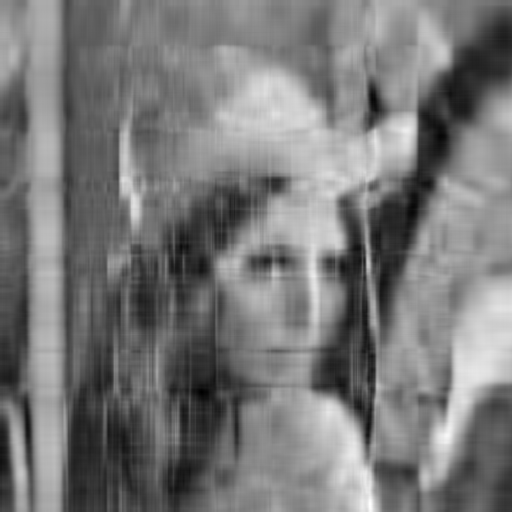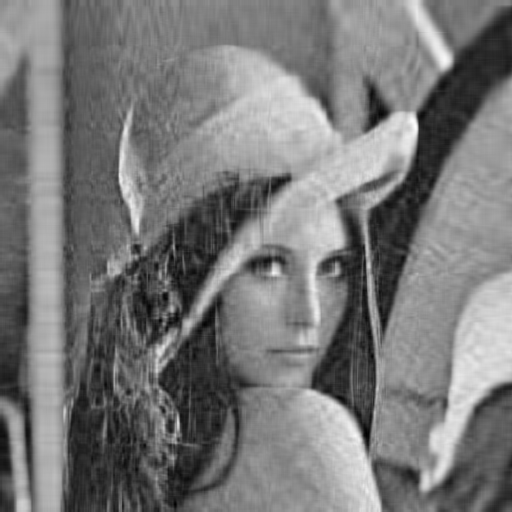## 代码

import cv2
import numpy as np
from numpy import linalg as la  # 用到别名
from scipy.misc import imsave

import scipy
print(im.shape)
gray = cv2.cvtColor(im, cv2.COLOR_BGR2GRAY)
U, Sigma, VT = la.svd(gray)
print('矩阵U的形状：', U.shape, '    矩阵Sigma的形状：',
Sigma.shape, '    矩阵VT的形状：', VT.shape)
se = np.eye(512, dtype=np.float64)
n = 512
i = 0
k = 30  # 保留特征值数目
# 改变特征值
while i < n:
if i > k - 1:
Sigma[i] = 0
se[i, i] = Sigma[i]
i += 1

svt = np.dot(se, VT)
usvt = np.dot(U, svt)
imsave('USVT_Sigma=30m.bmp', usvt)

# 参考资料

1. 秦川, 李小飞. 方阵的秩与特征值的关系[J]. 课程教育研究:学法教法研究, 2015(27):120-120.
2. 如何通俗易懂地解释「协方差」与「相关系数」的概念？
3. 如何理解矩阵特征值？马同学的回答
4. 矩阵的特征值分解与奇异值分解的几何意义
5. 杜美华, 孙建英. 正交变换的几何意义及其应用[J]. 哈尔滨师范大学自然科学学报, 2014, 30(3):36-39.

2016-01-16 16:38:16 baimafujinji 阅读数 12033
• ###### MATLAB图像处理

全面系统的学习MATLAB在图像处理中的应用

19905 人正在学习 去看看 魏伟

http://blog.csdn.net/baimafujinji/article/details/48467225

2.3.6 希尔伯特空间